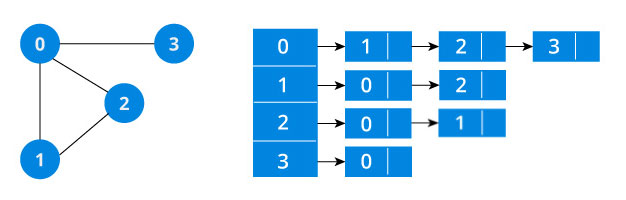An adjacency list represents a graph as an array of linked list.

The index of the array represents a vertex and each element in its linked list represents the other vertices that form an edge with the vertex.

A graph and its equivalent adjacency list representation is shown below.An adjacency list is efficient in terms of storage because we only need to store the values for the edges. For a sparse graph with millions of vertices and edges, this can mean a lot of saved space.

The simplest adjacency list needs a node data structure to store a vertex and a graph data structure to organize the nodes.

We stay close to the basic definition of graph - a collection of vertices and edges `{V, E}`. For simplicity we use an unlabeled graph as opposed to a labeled one i.e. the vertexes are identified by their indices 0,1,2,3.

Let's dig into the data structures at play here.

``````struct node
{
int vertex;
struct node* next;
};

struct Graph
{
int numVertices;
};``````

Don't let the `struct node** adjLists` overwhelm you.

All we are saying is we want to store a pointer to `struct node*`. This is because we don't know how many vertices the graph will have and so we cannot create an array of Linked Lists at compile time.

It is the same structure but by using the in-built list STL data structures of C++, we make the structure a bit cleaner. We are also able to abstract the details of the implementation.

``````class Graph
{
int numVertices;

public:
Graph(int V);
};``````

We use Java Collections to store the Array of Linked Lists.

``````class Graph
{
private int numVertices;
}``````

The type of LinkedList is determined what data you want to store in it. For a labeled graph, you could store a dictionary instead of an Integer

There is a reason Python gets so much love. A simple dictionary of vertices and its edges is a sufficient representation of a graph. You can make the vertex itself as complex as you want.

``````graph = {'A': set(['B', 'C']),
'B': set(['A', 'D', 'E']),
'C': set(['A', 'F']),
'D': set(['B']),
'E': set(['B', 'F']),
'F': set(['C', 'E'])}``````

## Adjacency List Code in C

``````#include <stdio.h>
#include <stdlib.h>

struct node
{
int vertex;
struct node* next;
};
struct node* createNode(int);

struct Graph
{
int numVertices;
};

struct Graph* createGraph(int vertices);
void addEdge(struct Graph* graph, int src, int dest);
void printGraph(struct Graph* graph);

int main()
{
struct Graph* graph = createGraph(6);

printGraph(graph);

return 0;
}

struct node* createNode(int v)
{
struct node* newNode = malloc(sizeof(struct node));
newNode->vertex = v;
newNode->next = NULL;
return newNode;
}

struct Graph* createGraph(int vertices)
{
struct Graph* graph = malloc(sizeof(struct Graph));
graph->numVertices = vertices;

graph->adjLists = malloc(vertices * sizeof(struct node*));

int i;
for (i = 0; i < vertices; i++)

return graph;
}

void addEdge(struct Graph* graph, int src, int dest)
{
// Add edge from src to dest
struct node* newNode = createNode(dest);

// Add edge from dest to src
newNode = createNode(src);
}

void printGraph(struct Graph* graph)
{
int v;
for (v = 0; v < graph->numVertices; v++)
{
printf("\n Adjacency list of vertex %d\n ", v);
while (temp)
{
printf("%d -> ", temp->vertex);
temp = temp->next;
}
printf("\n");
}
}``````

## Adjacency List code in C++

``````#include <iostream>
#include <list>
using namespace std;

class Graph
{
int numVertices;

public:
Graph(int V);
};

Graph::Graph(int vertices)
{
numVertices = vertices;
}

{
}

int main()
{
Graph g(4);

return 0;
}``````

``````import java.io.*;
import java.util.*;

class Graph
{
private int numVertices;

Graph(int vertices)
{
numVertices = vertices;

for (int i = 0; i < vertices; i++)
}

{